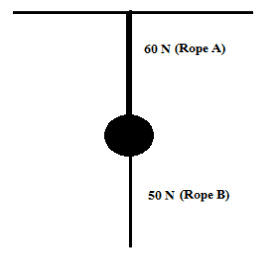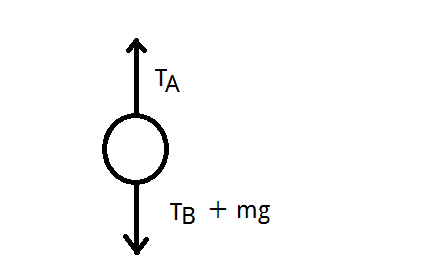## Homework Statement[/B]
A body is connected at the middle with two different ropes as in the figure. The maximum force that rope A can resist is 60 N, and for rope B its 50 N. What will happen if someone apply a downward force at the loose end of rope B, a) gradually b) very fast

## The Attempt at a Solution

My opinion is: if the force is greater than 50N, rope B will tear for both cases. Nothing will happen to rope A.

PeroK
Homework Helper
Gold Member
2021 Award
Assuming the diagram represents a vertical configuration, the mass of the body is relevant.

When the applied force is is gradual the tensions in both the strings have to be identical and equal to the applied force, if we neglect the mass of the body, as the body remains at rest in a permanent fashion. So as F increase it will reach 50 N first and then become 60 N so lower rope will break first. I am not able to think about the sudden application of force.

Last edited:
I think in second case also the lower rope will break first. if weight of the body cannot be neglected, then when you apply force even if suddenly the two tensions have to be equal but the lower rope may have less tension at any instant as in the beginning lower rope has zero tension and the tension is upper rope is equal to the weight of the body. So it is possible that at some instance above is 60 N and the lower one is below 50 N, in that case the upper rope will break.

Assuming the diagram represents a vertical configuration, the mass of the body is relevant.
The mass was not given in the problem.

PeroK
Homework Helper
Gold Member
2021 Award
If the mass is 7kg, say, then the upper rope will break without any additional force being applied.

If it's 5kg, then the upper rope will break when the applied force reaches about 12N.

If the mass is 7kg, say, then the upper rope will break without any additional force being applied.

If it's 5kg, then the upper rope will break when the applied force reaches about 12N.
Then I guess the mass is negligible.

PeroK
Homework Helper
Gold Member
2021 Award
Then I guess the mass is negligible.

Then you essentially have two ropes joined together and the lesser rope will always break first.

What about the case where the mass is 5kg and you apply a force of,say, 100N instantaneously?

How is the force transmitted to the upper rope?

Then you essentially have two ropes joined together and the lesser rope will always break first.

What about the case where the mass is 5kg and you apply a force of,say, 100N instantaneously?

How is the force transmitted to the upper rope?
Won't rope B break?

PeroK
Homework Helper
Gold Member
2021 Award
Won't rope B break?

That's what you have to work out. You need a free body diagram.

That's what you have to work out. You need a free body diagram.
I am confused about how the force will be transmitted.

Hi arpon ,

In part b) , when the downward force is applied very fast , will the acceleration of the mass/body be large or small ?

Hi arpon ,

In part b) , when the downward force is applied very fast , will the acceleration of the mass/body be large or small ?
Will the mass be accelarated? Won't the rope break if the force exceeds the limit of 50N?

Will the mass be accelarated?

Won't a large sudden force try to accelerate the bottom rope ? Isn't rope connected to the mass ? Won't the string and rope have same acceleration till the time they are connected ?

Won't the rope break if the force exceeds the limit of 50N?

This is what we are trying to find .

•arpon
PeroK
Homework Helper
Gold Member
2021 Award
I am confused about how the force will be transmitted.
It's perhaps not a good question. My thinking is:

1) the maximum tension in A is the tension in B plus ##mg##. If ##mg## is less than 10N then B must break first.

2) If ##mg## is greater than 10N then under a gradual increase in force, A must break first. But, under an instantaneous force it's not so clear what happens. If you assume that the system is rigid and the tension travels almost infinitely fast, then A will break.

Edit: Corrected A and B.

Last edited:
It's perhaps not a good question. My thinking is:

1) the maximum tension in B is the tension in A plus ##mg##. If ##mg## is less than 10N then A must break first.

2) If ##mg## is greater than 10N then under a gradual increase in force, B must break first. But, under an instantaneous force it's not so clear what happens. If you assume that the system is rigid and the tension travels almost infinitely fast, then B will break.I think the maximum tension in B is the tension in A minus ##mg##.

PeroK